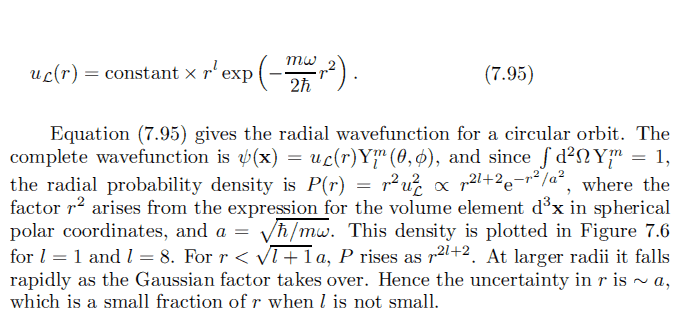# 3D Harmonic Oscillator Circular Orbit

## Homework Statement

I found this in Binney's text, pg 154 where he described the radial probability density ##P_{(r)} \propto r^2 u_L#### The Attempt at a Solution

Isn't the radial probability density simply the square of the normalized wavefunction, |ψ(x)|2? Why is there an additional factor of r2?

## Answers and Replies

When you integrate a function over a volume in spherical co-ordinates you integrate over r2sin(θ)drdθdø . The sin(θ)dθdø goes into the angular function and the r2dr into the radial function. I believe this is where it comes from.

•1 person
When you integrate a function over a volume in spherical co-ordinates you integrate over r2sin(θ)drdθdø . The sin(θ)dθdø goes into the angular function and the r2dr into the radial function. I believe this is where it comes from.

Probability = ##<\psi|\psi> = \int \psi^*\psi d^3r = \int \psi^*\psi r^2 dr d\Omega##

Where the wavefunction corresponding to the ket ##|\psi>## is ##u_L Y_l^m##

I think that's right.

Last edited:
Probability = ##<\psi|\psi> = \int \psi^*\psi d^3r = \int \psi^*\psi r^2 dr d\Omega##

Where the wavefunction corresponding to the ket ##|\psi>## is ##u_L Y_l^m##

I think that's right.

Correct.